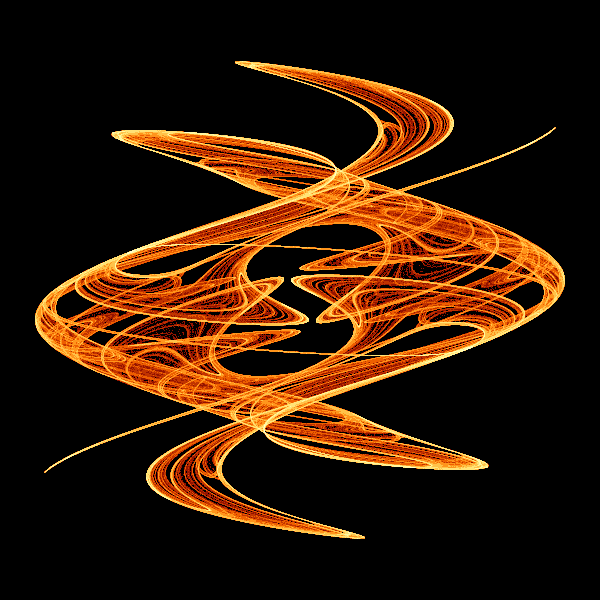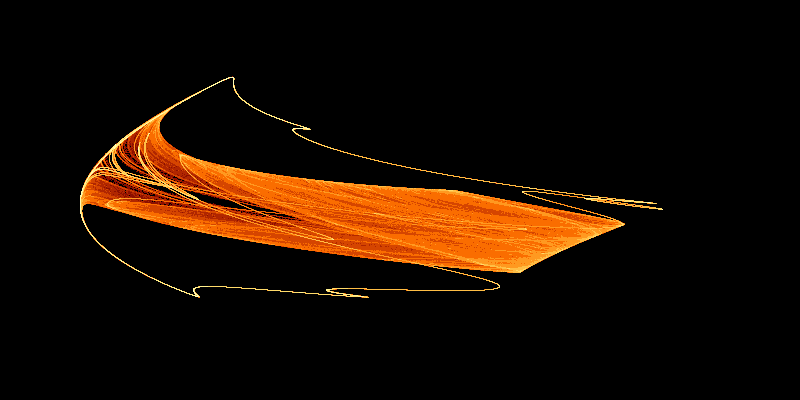# King's dream fractal with generativepy

Martin McBride, 2020-10-30
Tags kings dream fractal
Categories generativepy generative art

generativepy has moved to the pythoninformer.com website, and this page might not be up to date. Please visit the new location.

The king's dream fractal is based on an iterated function. Here we will look at an implementation using generativepy.

## Kings dream formula

The fractal equations for king's dream are:

```xnext = math.sin(A*x)+B*math.sin(A*y)
ynext = math.sin(C*x)+D*math.sin(C*y)
```

Where:

```A = 2.879879
B = -0.765145
C = -0.966918
D = 0.744728
```

We iterate over these functions, many times, each time feeding the new values for `xnext`, `ynext` back into the formula to calculate the next values. With initial values of `x = y = 2`, the first 10 iterations are:

```-0.11739262324428473 -1.6310096004414465
-1.0967288300305713 0.8579788756359019
-0.4587215217592031 0.3232191798205215
-1.5827141515778989 0.20016830786460135
0.5710597344430024 0.8559412049637697
0.5181954109793399 -1.0728971299066665
1.0365244305942398 0.1609266555576625
-0.18615820440184705 -0.9580907496626859
-0.22528068858502198 0.774409290433108
-1.208951509757061 -0.2908664982086804
```

These values at first appear to jump around fairly randomly. In the king's dream algorithm, for each value in the list we set the corresponding pixel to white (against a black background). After 100,000 iterations, the image looks like this:## The code

Here is the full code for the image above:

```from generativepy.bitmap import make_bitmap, Scaler
from generativepy.color import Color
import numpy as np
import math

MAX_COUNT = 100000
BLACK = Color(0)
WHITE = Color(1)
A = 2.879879
B = -0.765145
C = -0.966918
D = 0.744728

def paint(image, pixel_width, pixel_height, frame_no, frame_count):
scaler = Scaler(pixel_width, pixel_height, width=4, startx=-2, starty=-2)

counts = np.zeros([pixel_height, pixel_width], np.int32)

x = 2
y = 2
for i in range(MAX_COUNT):
x, y = math.sin(A*x)+B*math.sin(A*y), math.sin(C*x)+D*math.sin(C*y)
px, py = scaler.user_to_device(x, y)
counts[py, px] += 1

for px in range(pixel_width):
for py in range(pixel_height):
col = BLACK if counts[py, px]==0 else WHITE
image.putpixel((px, py), col.as_rgb_bytes())

make_bitmap('kings-dream-mono.png', paint, 600, 600)
```

We are using `generativepy.bitmap.make_bitmap` to make the image, which uses a `paint` function to do the actual drawing an a Pillow `Image` object.

We create a `Scaler` object that maps the 600 pixel square image onto a user space the is 4 units square, starting at (-2, -2) - that is, the user space origin is in the centre of the image.

The next thing we do is create an array to store which pixels have been marked by the fractal. We create a numpy array `counts` that is the same size as the output image. Generally a numpy array stores data in row-column order, so we make the array size `pixel_height` by `pixel_width`. The array is of type integer, because we will count the number of times each pixel is "hit".

Then we enter a loop where we iterate the equation `MAX_COUNT` times:

```    for i in range(MAX_COUNT):
x, y = math.sin(A*x)+B*math.sin(A*y), math.sin(C*x)+D*math.sin(C*y)
px, py = scaler.user_to_device(x, y)
counts[py, px] += 1
```

Each time through the loop we generate a new `(x, y)` value, which will be a floating point value that is within our user space, so in the square that is bounded by +/- 2.0 in the x and y dimensions. We use the `scaler` to convert user space to an image pixel value (integer coordinates in the range 0 to 599).

For each iteration, we add 1 to the corresponding element in the `counts` array.

Finally we fill out our image:

```    for px in range(pixel_width):
for py in range(pixel_height):
col = BLACK if counts[py, px]==0 else WHITE
image.putpixel((px, py), col.as_rgb_bytes())
```

We loop over every image pixel. If the equivalent element in the `counts` element is zero, the pixel is not part of the fractal and is set to black. If the pixel is greater than zero, the pixel is part of the fractal and is set to white.

## Improving the image

The image above is black and white. It shows which pixels have been touched by the fractal, but it doesn't tell the whole story. If we looked at the `counts` array we would see that different pixels have different counts. Some pixels have been visited once by the fractal, some have been visited more, perhaps many times. Certain parts of the space have a greater density of hits, and if we represent this with colour we will see more detail and a more interesting image.

Here is the image we will make:We will make several code changes. The first is to increase the `MAX_COUNT` value to 10 million. That sounds a lot, but it won't take too long on a modern PC. This will create a more detailed image.

Remember that we are iterating over the functions to generate a series of `(x, y)` values and counting how many times each indvidual pixel gets hit. There will be a lot of pixels that have a count of 0. These are the black areas of the image which are not part of the fractal - you could loop forever and these pixels will never get a single hit. The remaining pixels have hits counts between one and several thousand.

However, the distribution of counts is not even. Thge vast majority of pixels have very low counts, and tiny number have the highest counts. If we assigned the pixel colours as a linear function of count, most of the pixels would have very similar colours, so we would miss a lot of detail.

One solution is to apply a logarithm function to the counts. This ensures that the range 1 to 10 has the same amount of colour variation as the range 10 to 100, the range 100 to 1000 and the range 1000 to 10,000. Here is the code:

```    counts = np.log(counts + 1).astype(np.int)
```

We add 1 because `log(0)` is undefined. Since `log(1)` is zero, this means that any pixels that had a raw count value zero will remain at zero after the conversion. `counts` now contains the log of the raw counts. We then construct a colour table for values from 0 to the largest value in `counts`:

```    max_count = np.max(counts)
colors = build_color_table(max_count+1)
```

The colour table is constructed like this:

```def build_color_table(size):
table = [Color.of_hsl(0.2*i/size, 1, 0.2+0.8*i/size) for i in range(size)]
table = BLACK
return table
```

The hue value varies from 0 t0 0.2, which covers red to orange to yellow. The lightness goes from 0.2 to 1.0, which covers dark to normal to full white. This gives and attractive spread of colours. We specifically set the 0 element to black.

Finally we loop over every pixel in the image, setting the colour based on the `count` (which of course is the log o fteh raw count value). Here is the full code:

```from generativepy.bitmap import make_bitmap, Scaler
from generativepy.color import Color
import numpy as np
import math

MAX_COUNT = 10000000
BLACK = Color(0)
WHITE = Color(1)
A = 2.879879
B = -0.765145
C = -0.966918
D = 0.744728

def build_color_table(size):
table = [Color.of_hsl(0.2*i/size, 1, 0.2+0.8*i/size) for i in range(size)]
table = BLACK
return table

def paint(image, pixel_width, pixel_height, frame_no, frame_count):
scaler = Scaler(pixel_width, pixel_height, width=4, startx=-2, starty=-2)

counts = np.zeros([pixel_height, pixel_width], np.int32)

x = 2
y = 2
for i in range(MAX_COUNT):
x, y = math.sin(A*x)+B*math.sin(A*y), math.sin(C*x)+D*math.sin(C*y)
px, py = scaler.user_to_device(x, y)
counts[py, px] += 1

counts = np.log(counts + 1).astype(np.int)
max_count = np.max(counts)
colors = build_color_table(max_count+1)

for px in range(pixel_width):
for py in range(pixel_height):
col = colors[counts[py, px]]
image.putpixel((px, py), col.as_rgb_bytes())

make_bitmap('kings-dream.png', paint, 600, 600)
```

## Variants

You can try different values of the constants. `A` and `B` need to be in the range -3 tp +3, while `C` and `D` need to be in the range -1.5 to +1.5, otherwise the values wil fly off to infinity rather than creating a pattern.

Be aware that most numbers you choose will not create pleasing patterns. You will need to experiment to find something that looks nice, and then do even more fine tuning to get something really nice.

You can also try varying the function. You can replace `sin` with `cos` in some or all of the equations. This will give different but similar patterns. Here is an example:This uses functions:

```x, y = math.cos(A*x)+B*math.cos(A*y), math.cos(C*x)+D*math.sin(C*y)
```

With initial values:

```A = 2.5
B = -0.6
C = -0.9
D = 0.8
```

See the iterated functions article for a list of other fractal examples.